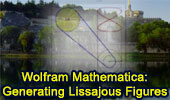# Generating Lissajous Figures with Wolfram Mathematica, Video and News

Generating Lissajous Figures with Wolfram Mathematica - Video

Video Description
"Generating Lissajous Figures" from The Wolfram Demonstrations Project
Contributed by: Michael Rogers (Oxford College/Emory University)
Source: Demonstrations.Wolfram.com

Generating Lissajous Figures
Given two wheels connected by a belt and a point on the outer rim of each, one can generate a Lissajous figure by the motion of the wheels. The coordinates of a point on a wheel can be described by sine functions of a certain frequency and phase shift.

Wolfram Demonstrations Project
Conceived by Mathematica creator and scientist Stephen Wolfram as a way to bring computational exploration to the widest possible audience, the Wolfram Demonstrations Project is an open-code resource that uses dynamic computation to illuminate concepts in science, technology, mathematics, art, finance, and a remarkable range of other fields. Source: Demonstrations.Wolfram.com